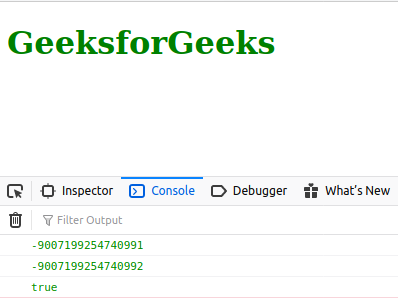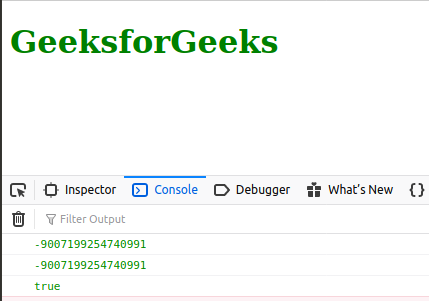# JavaScript Number.MIN_SAFE_INTEGER Constant

Below is the example of the Number.MIN_SAFE_INTEGER Constant.

• Example:

 `<``script` `type``=``"text/javascript"``> ` `    ``document.write(Number.MIN_SAFE_INTEGER);  ` `  `

• Output:

`-9007199254740991`

The JavaScript Number.MIN_SAFE_INTEGER is a constant number which represents the minimum safe integer. This constant has a value of (-(253 – 1)).

Use Number.MIN_SAFE_INTEGER, as a property of a number object because it is a static property of number.

Syntax:

`Number.MIN_SAFE_INTEGER`

Return Value: Constant number.

Example 1: Below example illustrates the use of simple Number.MIN_SAFE_INTEGER constant.

 ` ` ` `  `<``html` `lang``=``"en"``> ` ` `  `<``body``> ` `    ``<``h1` `style``=``"color: green;"``>GeeksforGeeks ` `    ``<``script` `type``=``"text/javascript"``> ` `        ``const a = Number.MIN_SAFE_INTEGER - 1; ` `        ``const b = Number.MIN_SAFE_INTEGER - 2; ` ` `  `        ``console.log(Number.MIN_SAFE_INTEGER); ` `        ``console.log(a); ` `        ``console.log(a === b); ` `    `` ` ` ` ` `  ` `

Output:Example 2: Below example illustrates the usage of Number.MIN_SAFE_INTEGER constant using Math.pow() function.

 ` ` ` `  `<``html` `lang``=``"en"``> ` ` `  `<``body``> ` `    ``<``h1` `style``=``"color: green;"``>GeeksforGeeks ` `    ``<``script` `type``=``"text/javascript"``> ` `        ``const c = Number.MIN_SAFE_INTEGER; ` `        ``const d = -(Math.pow(2, 53) - 1); ` ` `  `        ``console.log(c); ` `        ``console.log(d); ` `        ``console.log(c === d); ` `    `` ` ` ` ` `  ` `

Output:Note: Internet explorer is not supported.

Supported Browsers:

1. Chrome
2. Edge
3. Firefox
4. Opera
5. SafariMy Personal Notes arrow_drop_upCheck out this Author's contributed articles.

If you like GeeksforGeeks and would like to contribute, you can also write an article using contribute.geeksforgeeks.org or mail your article to contribute@geeksforgeeks.org. See your article appearing on the GeeksforGeeks main page and help other Geeks.

Please Improve this article if you find anything incorrect by clicking on the "Improve Article" button below.